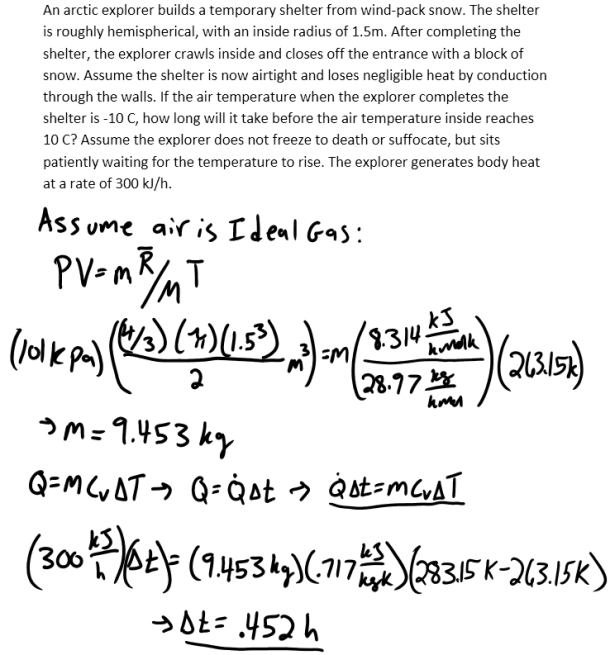heat and mass transfer problems engineering equations heat and mass transfer school homework engineering heat and mass transfer formulas heat and mass transfer solutions to heat and mass transfer problems full solution engineering problem solution heat and mass transfer math problems engineering equations heat and mass transfer school homework engineering solutions to heat and mass transfer formulas heat problems mass problem solutions to transfer problems full solution heat and mass transfer
heat and mass transfer problems engineering equations heat and mass transfer school homework engineering heat and mass transfer formulas heat and mass transfer solutions to heat and mass transfer problems full solution engineering problem solution heat and mass transfer math problems engineering equations heat and mass transfer school homework engineering solutions to heat and mass transfer formulas heat problems mass problem solutions to transfer problems full solution heat and mass transfer
Highalphabet Home Page heat and mass transfer problem solutions Heat and Mass Transfer Page
An arctic explorer builds a temporary shelter from wind-pack snow. The shelter is roughly hemispherical, with an inside radius of 1.5m. After completing the shelter, the explorer crawls inside and closes off the entrance with a block of snow. Assume the shelter is now airtight and loses negligible heat by conduction through the walls. If the air temperature when the explorer completes the shelter is -10 C, how long will it take before the air temperature inside reaches 10 C? Assume the explorer does not freeze to death or suffocate, but sits patiently waiting for the temperature to rise. The explorer generates body heat at a rate of 300 kJ/h.An arctic explorer builds a temporary shelter from wind-pack snow. The shelter is roughly hemispherical, with an inside radius of 1.5m. After completing the shelter, the explorer crawls inside and closes off the entrance with a block of snow. Assume the shelter is now airtight and loses negligible heat by conduction through the walls. If the air temperature when the explorer completes the shelter is -10 C, how long will it take before the air temperature inside reaches 10 C? Assume the explorer does not freeze to death or suffocate, but sits patiently waiting for the temperature to rise. The explorer generates body heat at a rate of 300 kJ/h.